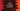# C program to check if a number is a perfect number or not### C program to check if a number is a perfect number or not :

In this tutorial, we will learn how to find if a number is perfect or not by using C programming language. A positive number is called a perfect number if the sum of its proper positive divisors is equal to the number. For example, 6 is a perfect number. Because : Its proper divisors from 1 to 5 are 1, 2, and 3. Sum is 1 + 2 + 3 = 6 which is equal to the number itself. So, it is a perfect number. Let’s take a look at the program :

### C program :

``````#include

int main(){
//1
int no;
int sum = 0;
int divisor = 1;

//2
printf("Enter the number : ");
scanf("%d",&no);

//3
for(divisor = 1;divisor``````

### Explanation :

The commented numbers in the above program denote the step number below :

1. Create one integer variable no to store the number, one variable sum to store the sum of all perfect divisors and one more variable divisor that indicate the current divisor.
2. Ask the user to enter a number. Read it and save it in variable no.
3. Run one for-loop. This loop will run from divisor = 1 to divisor = no - 1.
4. Check if the current divisor is perfect-divisor or not, i.e. if the divisor divides the number, the remainder should be 0. If it is a perfect-divisor, add its value to sum.
5. Finally, check if the sum is equal to the original number or not. If yes, it is a perfect number, else it is not. Print out the message to the user.

### Sample Output :

``````Enter the number : 567
567 is not a perfect number

Enter the number : 6
6 is a perfect number

Enter the number : 8128
8128 is a perfect number

Enter the number : 496
496 is a perfect number

Enter the number : 28
28 is a perfect number``````

### Where is the color and why codevscolor ?

Long story short, I love paintings and I paint on weekends. We(me and my wife) have one Youtube channel. Don't forget to subscribe ❤️❤️🤩